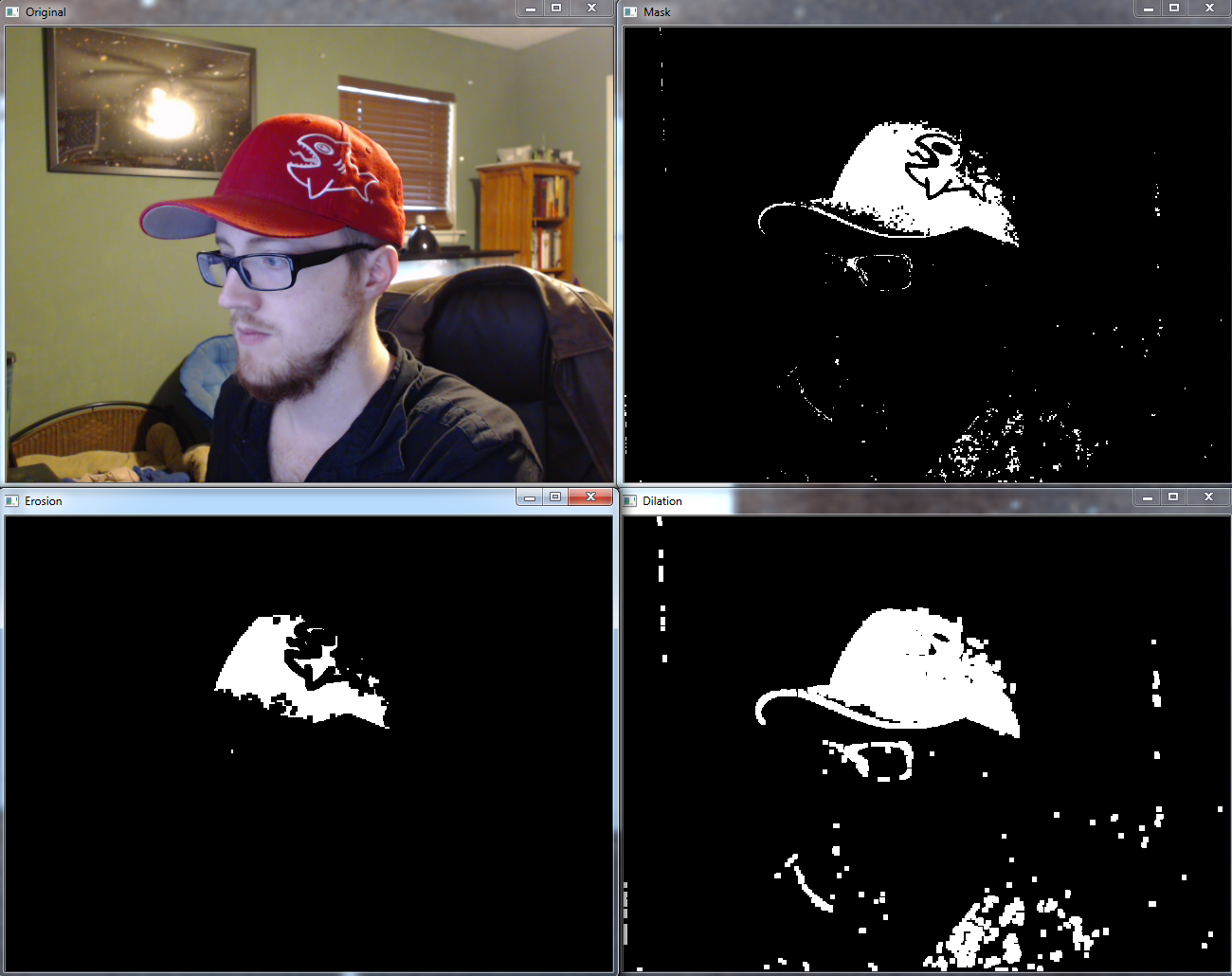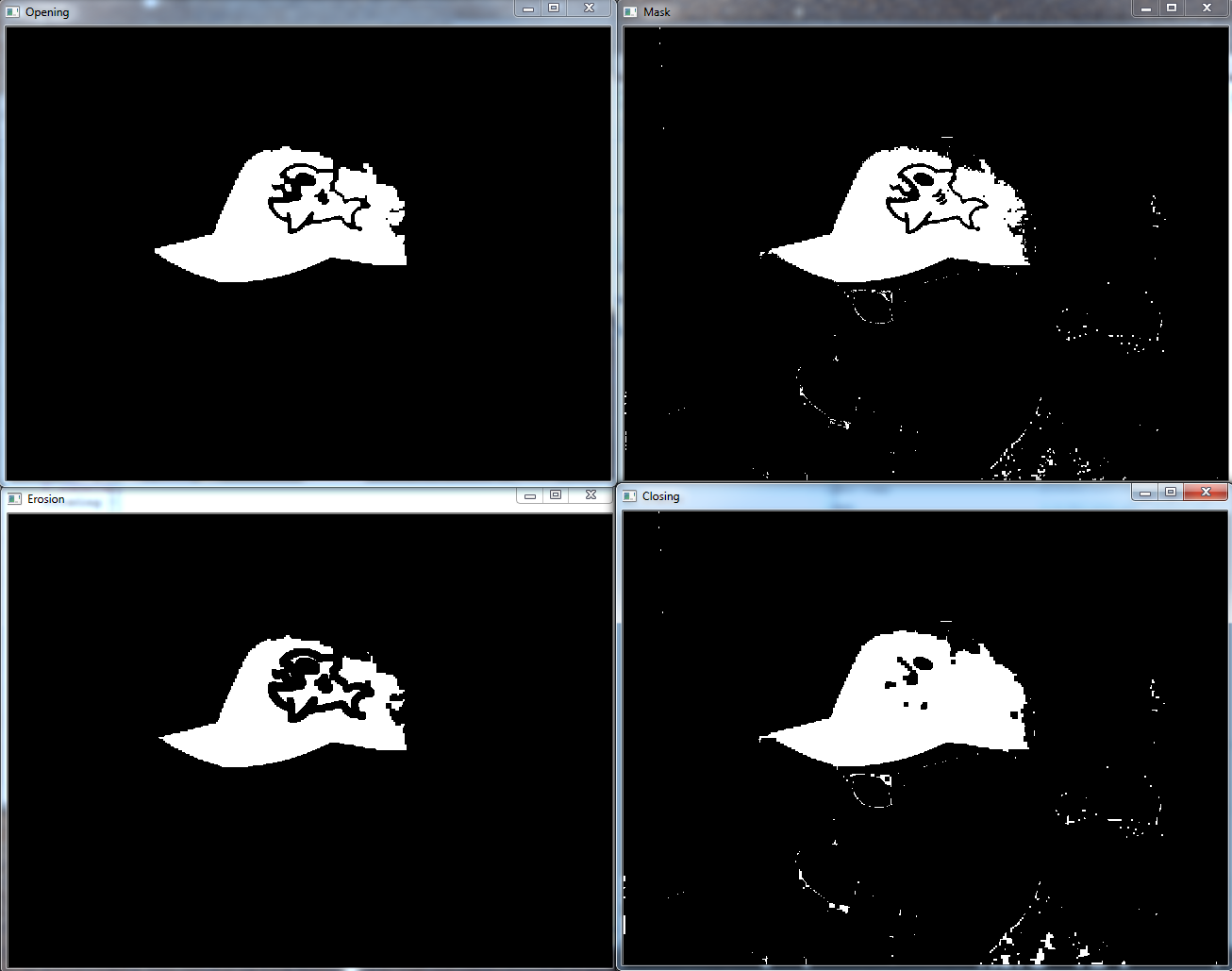Morphological Transformations OpenCV Python Tutorial

In this OpenCV with Python tutorial, we're going to cover Morphological Transformations. These are some simple operations that we can perform based on the image's shape.

These tend to come in pairs. The first pair we're going to talk about is Erosion and Dilation. Erosion is where we will "erode" the edges. The way these work is we work with a slider (kernel). We give the slider a size, let's say 5 x 5 pixels. What happens is we slide this slider around, and if all of the pixels are white, then we get white, otherwise black. This may help eliminate some white noise. The other version of this is Dilation, which basically does the opposite: Slides around, if the entire area isn't black, then it is converted to white. Here's an example:

import cv2
import numpy as np

cap = cv2.VideoCapture(0)

while(1):

hsv = cv2.cvtColor(frame, cv2.COLOR_BGR2HSV)

lower_red = np.array([30,150,50])
upper_red = np.array([255,255,180])

kernel = np.ones((5,5),np.uint8)

cv2.imshow('Original',frame)
cv2.imshow('Erosion',erosion)
cv2.imshow('Dilation',dilation)

k = cv2.waitKey(5) & 0xFF
if k == 27:
break

cv2.destroyAllWindows()
cap.release()

Result:The next pair is "opening" and "closing." The goal with opening is to remove "false positives" so to speak. Sometimes, in the background, you get some pixels here and there of "noise." The idea of "closing" is to remove false negatives. Basically this is where you have your detected shape, like our hat, and yet you still have some black pixels within the object. Closing will attempt to clear that up.

cap = cv2.VideoCapture(1)

while(1):

hsv = cv2.cvtColor(frame, cv2.COLOR_BGR2HSV)

lower_red = np.array([30,150,50])
upper_red = np.array([255,255,180])

kernel = np.ones((5,5),np.uint8)

cv2.imshow('Original',frame)
cv2.imshow('Opening',opening)
cv2.imshow('Closing',closing)

k = cv2.waitKey(5) & 0xFF
if k == 27:
break

cv2.destroyAllWindows()
cap.release()

The result here:Two other options that aren't really useful for our case here are "tophat" and "blackhat:"

# It is the difference between input image and Opening of the image
cv2.imshow('Tophat',tophat)

# It is the difference between the closing of the input image and input image.
cv2.imshow('Blackhat',blackhat)

In the next tutorial, we're going to be discussing image gradients and edge detection.

The next tutorial:• Drawing and Writing on Image OpenCV Python Tutorial

• Image Operations OpenCV Python Tutorial

• Image arithmetics and Logic OpenCV Python Tutorial

• Thresholding OpenCV Python Tutorial

• Color Filtering OpenCV Python Tutorial

• Blurring and Smoothing OpenCV Python Tutorial

• Morphological Transformations OpenCV Python Tutorial
• Canny Edge Detection and Gradients OpenCV Python Tutorial

• Template Matching OpenCV Python Tutorial

• GrabCut Foreground Extraction OpenCV Python Tutorial

• Corner Detection OpenCV Python Tutorial

• Feature Matching (Homography) Brute Force OpenCV Python Tutorial

• MOG Background Reduction OpenCV Python Tutorial

• Haar Cascade Object Detection Face & Eye OpenCV Python Tutorial